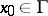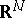# Jordan theorem

(diff) ← Older revision | Latest revision (diff) | Newer revision → (diff)
A plane simple closed curvedecomposes the planeinto two connected components and is their common boundary. Established by C. Jordan . Together with the similar assertion: A simple arc does not decompose the plane, this is the oldest theorem in set-theoretic topology.
Of the two components, one (the inside of) is bounded; it is characterized by the fact that the order of every point in it with respect tois; the other (the outside of) is unbounded, and the orders of its points with respect toare zero. For any pointof the bounded componentand every point, there exists a simple arc with endsandand all points of which, except, are contained in(Schoenflies' theorem).
The Jordan (curve) theorem can be generalized according to the dimension: Every-dimensional submanifold ofhomeomorphic to a sphere decomposes the space into two components and is their common boundary; forthis was proved by e dimension','../l/l057830.htm','Lebesgue function','../l/l057840.htm','Lebesgue inequality','../l/l057850.htm','Lebesgue integral','../l/l057860.htm','Lebesgue measure','../l/l057870.htm','Lebesgue summation method','../l/l057940.htm','Lebesgue theorem','../l/l057950.htm','Measure','../m/m063240.htm','Metric space','../m/m063680.htm','Metric theory of functions','../m/m063700.htm','Orthogonal series','../o/o070370.htm','Perron method','../p/p072370.htm','Potential theory','../p/p074140.htm','Regular boundary point','../r/r080680.htm','Singular integral','../s/s085570.htm','Suslin theorem','../s/s091480.htm','Urysohn–Brouwer lemma','../u/u095860.htm','Vitali variation','../v/v096790.htm')" style="background-color:yellow;">H. Lebesgue, and in the general case by L.E.J. Brouwer, whence the-dimensional theorem is sometimes called the Jordan–Brouwer theorem or Jordan–Brouwer separation theorem.